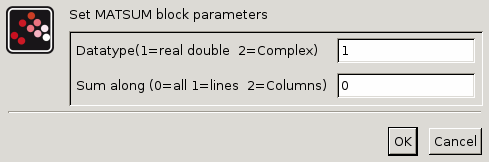Scilab Home page | Wiki | Bug tracker | Forge | Mailing list archives | ATOMS | File exchange
Change language to: Français - Português - 日本語 - Русский

See the recommended documentation of this function

Scilab help >> Xcos > palettes > Matrix operation palette > MATSUM

# MATSUM

Sum of Matrix's Elements

### Block Screenshot### Description

The MATSUM block returns the sum of the element of an input matrix/vector.

When the Sum along is set to:

• 0 (all) the block outputs the sum of all the elements of the matrix. The output is then a scalar.

• 1 (lines) the block is a row-wise sum. The output is a row vector.

• 2 (Columns) the block is a column-wise sum. The output is a column vector.

The equivalent function of this block in Scilab is sum.

### Dialog box• Datatype(1=real double 2=Complex)

It indicates the type of the output. It support only the two types double (1) and complex (2). If we input another entry in this label xcos will print the message "Datatype is not supported".

Properties : Type 'vec' of size 1.

• Sum along (0=all 1=lines 2=Columns)

Indicates the used rule to sum. For more information see the description part.

Properties : Type 'vec' of size 1.

### Default properties

• always active: no

• direct-feedthrough: yes

• zero-crossing: no

• mode: no

• regular inputs:

- port 1 : size [-1,-2] / type 1

• regular outputs:

- port 1 : size [1,1] / type 1

• number/sizes of activation inputs: 0

• number/sizes of activation outputs: 0

• continuous-time state: no

• discrete-time state: no

• object discrete-time state: no

• name of computational function: mat_sum

### Interfacing function

• SCI/modules/scicos_blocks/macros/MatrixOp/MATSUM.sci

### Computational function

• SCI/modules/scicos_blocks/src/c/mat_sum.c

• SCI/modules/scicos_blocks/src/c/mat_suml.c

• SCI/modules/scicos_blocks/src/c/mat_sumc.c

• SCI/modules/scicos_blocks/src/c/matz_sum.c

• SCI/modules/scicos_blocks/src/c/matz_suml.c

• SCI/modules/scicos_blocks/src/c/matz_sumc.c